# Form of an algebraic group

(diff) ← Older revision | Latest revision (diff) | Newer revision → (diff)defined over a fieldAn algebraic group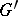defined overand isomorphic toover some extensionof. In this caseis called an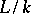-form of. Ifis the separable closure ofin a fixed algebraically closed ground field(a universal domain), then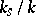-forms are simply called-forms of. Two-forms of a group are said to be equivalent if they are isomorphic over. The set of equivalence classes of-forms ofis denoted by(in the caseby) (see , , ).

Example. Let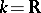,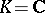. Then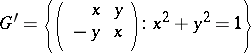andare two subgroups of the general linear group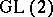defined over, andis a-form of(the isomorphism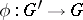, defined over, is given by the formula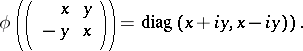This-form is not equivalent to(if one regardsas a-form of itself relative to the identity isomorphism). In this example, the setconsists of the two elements represented by the two-forms above.

The problem of classifying forms of algebraic groups can be naturally reformulated in the language of Galois cohomology, , . Namely, suppose thatis a Galois extension with Galois group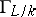(equipped with the Krull topology). The groupacts naturally on the group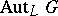of all-automorphisms of, and also on the set of all-isomorphisms fromto(in coordinates, these actions reduce to applying the automorphisms into the coefficients of the rational functions defining the respective mappings). Letbe some-isomorphism, let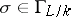and let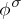be the image ofunder the action of. Then the mapping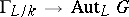,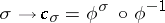, is a continuous-cocycle ofwith values in the discrete group. When replacingby another-isomorphism, this cocycle changes to a cocycle in the same cohomology class. Thus arises a mapping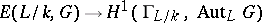. The main importance of the cohomological interpretation of the forms ofconsists in the fact that this mapping is bijective. In the case when all automorphisms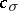are inner,is called an inner form of, and otherwise an outer form.

For connected reductive groups there is a thoroughly developed theory of forms, where relative versions of the structure theory of linear algebraic groups over an algebraically closed field are established:-roots, the-Weyl group, the Bruhat decomposition over, etc. Here the role of maximal tori is played by maximal-split tori, and that of Borel subgroups by minimal-parabolic subgroups , , , . This theory enables one to reduce the question of classifying forms to that of classifying anisotropic reductive groups over(see Anisotropic group; Anisotropic kernel). The question of classifying the latter depends essentially on the properties of the field. Ifand, then the characterization of forms of semi-simple algebraic groups is the same as that of real forms of complex semi-simple algebraic groups (see Complexification of a Lie group).

How to Cite This Entry:
Form of an algebraic group. Encyclopedia of Mathematics. URL: http://encyclopediaofmath.org/index.php?title=Form_of_an_algebraic_group&oldid=17664
This article was adapted from an original article by V.L. Popov (originator), which appeared in Encyclopedia of Mathematics - ISBN 1402006098. See original article